# quantum field theory

(redirected from quantum field theories)
Also found in: Dictionary, Thesaurus.
Related to quantum field theories: Relativistic quantum field theory

## quantum field theory,

study of the quantum mechanical interaction of elementary particleselementary particles,
the most basic physical constituents of the universe. Basic Constituents of Matter

Molecules are built up from the atom, which is the basic unit of any chemical element. The atom in turn is made from the proton, neutron, and electron.
and fieldsfield,
in physics, region throughout which a force may be exerted; examples are the gravitational, electric, and magnetic fields that surround, respectively, masses, electric charges, and magnets. The field concept was developed by M.
. Quantum field theory applied to the understanding of electromagnetism is called quantum electrodynamicsquantum electrodynamics
(QED), quantum field theory that describes the properties of electromagnetic radiation and its interaction with electrically charged matter in the framework of quantum theory.
(QED), and it has proved spectacularly successful in describing the interaction of light with matter. The calculations, however, are often complex. They are usually carried out with the aid of Feynman diagrams (named after American physicist Richard P. FeynmanFeynman, Richard Phillips
, 1918–88, American physicist, b. New York City, B.S. Massachusetts Institute of Technology, 1939, Ph.D. Princeton, 1942. From 1942 to 1945 he worked on the development of the atomic bomb.
), simple graphs that represent possible variations of interactions and provide an elegant shorthand for precise mathematical equations. Quantum field theory applied to the understanding of the strong interactionsstrong interactions,
actions between elementary particles mediated, or carried, by gluons. They are responsible for the binding of protons and neutrons in the nucleus and interactions between quarks.
between quarks and between protonsproton,
elementary particle having a single positive electrical charge and constituting the nucleus of the ordinary hydrogen atom. The positive charge of the nucleus of any atom is due to its protons.
, neutronsneutron,
uncharged elementary particle of slightly greater mass than the proton. It was discovered by James Chadwick in 1932. The stable isotopes of all elements except hydrogen and helium contain a number of neutrons equal to or greater than the number of protons.
, and other baryonsbaryon
[Gr.,=heavy], class of elementary particles that includes the proton, the neutron, and a large number of unstable, heavier particles, known as hyperons. From a technical point of view, baryons are strongly interacting fermions; i.e.
and mesonsmeson
[Gr.,=middle (i.e., middleweight)], class of elementary particles whose masses are generally between those of the lepton class of lighter particles and those of the baryon class of heavier particles. From a technical point of view mesons are strongly interacting bosons; i.
is called quantum chromodynamicsquantum chromodynamics
(QCD), quantum field theory that describes the properties of the strong interactions between quarks and between protons and neutrons in the framework of quantum theory.
(QCD); QCD has a mathematical structure similar to that of QED.

### Bibliography

See R. P. Feynman, QED (1985); F. J. Yndurain, The Theory of Quark and Gluon Interactions (1993).

## Quantum field theory

The quantum-mechanical theory of physical systems whose dynamical variables are local functions of space and time. As distinguished from the quantum mechanics of atoms, quantum field theories describe systems with an infinite number of degrees of freedom. Such theories provide the natural language for describing the interactions and properties of elementary particles, and have proved to be successful in providing the basis for the fundamental theories of the interactions of matter. The present understanding of the basic forces of nature is based on quantum field theories of the strong, weak, electromagnetic, and gravitational interactions. Quantum field theory is also useful in the study of many-body systems, especially in situations where the characteristic length of a system is large compared to its microscopic scale. See Quantum mechanics

Quantum field theory originated in the attempt, in the late 1920s, to unify P. A. M. Dirac's relativistic electron theory and J. C. Maxwell's classical electrodynamics in a quantum theory of interacting photon and electron fields. This effort was completed in the 1950s and was extremely successful. At present the quantitative predictions of the theory are largely limited to perturbative expansions (believed to be asymptotic) in powers of the fine-structure constant. However, because of the extremely small value of this parameter, α = e2/&planck;c &ap; 1/137 (where e is the electron charge, &planck; is Planck's constant divided by 2&pgr;, and c is the speed of light), such an expansion is quite adequate for most purposes. The remarkable agreement of the predictions of quantum electrodynamics with high-precision experiments (sometimes to an accuracy of 1 part in 1012) provides strong evidence for the validity of the basic tenets of relativistic quantum field theory. See Classical field theory, Electromagnetic radiation, Maxwell's equations, Perturbation (quantum mechanics), Photon, Quantum electrodynamics, Relativistic electrodynamics, Relativistic quantum theory

Quantum field theory also provides the natural framework for the treatment of the weak, strong, and gravitational interactions.

The first of such applications was Fermi's theory of the weak interactions, responsible for radioactivity, in which a hamiltonian was constructed to describe beta decay as a product of four fermion fields, one for each lepton or nucleon. This theory has been superseded by the modern electroweak theory that unifies the weak and the electromagnetic interactions into a common framework. This theory is a generalization of Maxwell's electrodynamics which was the first example of a gauge theory, based on a continuous local symmetry. In the case of electromagnetism the local gauge symmetry is the space-time-dependent change of the phase of a charged field. The existence of massless spin-1 particles, photons, is one of the consequences of the gauge symmetry. The electroweak theory is based on generalizing this symmetry to space-time-dependent transformations of the labels of the fields, based on the group SU(2) × U(1). However, unlike electromagnetism, part of this extended symmetry is not shared by the ground state of the system. This phenomenon of spontaneous symmetry breaking produces masses for all the elementary fermions and for the gauge bosons that are the carriers of the weak interactions, the W± and Z bosons. (This is known as the Higgs mechanism.) The electroweak theory has been confirmed by many precision tests, and almost all of its essential ingredients have been verified. See Electroweak interaction, Intermediate vector boson, Symmetry breaking, Symmetry laws (physics), Weak nuclear interactions

The application of quantum field theory to the strong or nuclear interactions dates from H. Yukawa's hypothesis that the short-range nuclear forces arise from the exchange of massive particles that are the quanta of local fields coupled to the nucleons, much as the electromagnetic interactions arise from the exchange of massless photons that are the quanta of the electromagnetic field. The modern theory of the strong interactions, quantum chromodynamics, completed in the early 1970s, is also based on a local gauge theory. This is a theory of spin-½ quarks invariant under an internal local SU(3) (color) gauge group. The observed hadrons (such as the proton and neutron) are SU(3) color-neutral bound states of the quarks whose interactions are dictated by the gauge fields (gluons). This theory exhibits almost-free-field behavior of quarks and gluons over distances and times short compared to the size of a hadron (asymptotic freedom), and a strong binding of quarks at large separations that results in the absence of colored states (confinement). See Elementary particle, Gluons, Meson, Quantum chromodynamics, Quarks

Quantum field theory has been tested down to distances of 10-20 m. There appears to be no reason why it should not continue to work down to Planck's length, (G&planck;/c3)1/2 &ap; 10-35 m (where G is the gravitational constant), where the quantum effects of gravity become important. In the case of gravity, A. Einstein's theory of general relativity already provides a very successful classical field theory. However, the union of quantum mechanics and general relativity raises conceptual problems that seem to call for a radical reexamination of the foundations of quantum field theory. See Fundamental interactions, Gravitation, Quantum gravitation, Relativity

McGraw-Hill Concise Encyclopedia of Physics. © 2002 by The McGraw-Hill Companies, Inc.
The following article is from The Great Soviet Encyclopedia (1979). It might be outdated or ideologically biased.

## Quantum Field Theory

the quantum theory of systems with an infinite number of degrees of freedom (physical fields). It took shape as a generalization of quantum mechanics in connection with the problem of describing the processes of production, absorption, and mutual transformations of elementary particles and later found extensive application in solid-state theory and the theory of the atomic nucleus. It is now the main theoretical method for studying quantum systems.

Duality of classical theory. In classical theory, the formation of which was basically complete by the early 20th century, the physical picture of the world consists of two elements, particles and fields. Particles are tiny bits of matter that move according to the laws of classical Newtonian mechanics. Each of them has three degrees of freedom: its position is defined by three coordinates, such as x, y, and z; if the dependence of the coordinates on time is known, exhaustive information on the particle’s motion is provided.

The description of fields is much more complicated. For example, to define an electric field means to give its intensity E at all points in space. Thus, to describe a field it is necessary to know not three quantities, as in the case of a particle, but rather an infinitely large number of quantities at each moment; in other words, a field has an infinite number of degrees of freedom. Naturally the laws of the dynamics of an electromagnetic field, which were determined primarily as a result of the studies of M. Faraday and J. Maxwell, prove to be more complicated than the laws of mechanics. This is the main, although not the only, difference between fields and particles: particles are discrete, whereas fields are continuous; an electromagnetic field (or electromagnetic waves) may be produced and absorbed, but appearance and disappearance are alien to the particles of classical mechanics; finally, electromagnetic waves may, by superposition, amplify or damp each other or even completely suppress each other (interference of waves), which does not, of course, take place during the superposition of streams of particles.

Although particles and waves are interwoven by a complex network of interactions, each acts as the carrier of fundamentally different individual features. Distinctive features of duality are inherent in the picture of the world in classical theory. The discovery of quantum phenomena replaced this picture with another, which may be called the dual picture.

Quanta of an electromagnetic field. In 1900, M. Planck first introduced into physics the concept of a bundle, or quantum, of radiation to explain the principles of thermal radiation of bodies. The energy € of such a quantum is proportional to the frequency v of the radiated electromagnetic wave: ε = hv, where the proportionality factor is h = 6.62 × 10–27 erg • sec (this came to be called Planck’s constant). A. Einstein extended Planck’s idea of the discreteness of radiation, assuming that it is inherent in electromagnetic radiation itself rather than being associated with some special mechanism of interaction between radiation and matter. Electromagnetic radiation “consists” of such quanta, or photons. These concepts were confirmed by experiment—the principles of the photoelectric effect and the Compton effect were explained on their basis.

Thus, characteristics of discreteness that previously were ascribed only to particles are inherent in electromagnetic radiation. Like a particle (or corpuscle), a photon has a definite energy, momentum, and spin and always exists as a unified whole. In addition to corpuscular properties, however, the photon also has wave properties that are manifested in such phenomena as diffraction and interference of light. Therefore, it could be called a wave-particle.

Wave-particle duality. The dualistic wave-particle concept of the electromagnetic field quantum—the photon—was extended by L. de Broglie to all types of matter. According to de Broglie’s hypothesis, electrons, protons, and any other particles have not only corpuscular but also wave properties. This is manifested quantitatively in the de Broglie relations, which link such corpuscular quantities as the energy ε and momentum p of a particle with quantities characteristic of a wave description—the wavelength λ and the frequency v: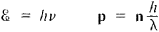where n is a unit vector indicating the direction of wave propagation.

Wave-particle duality, which was confirmed experimentally, required reexamination of the laws of motion and of the very methods of describing moving objects. Quantum mechanics (or wave mechanics) took shape. Its most important feature is the idea of giving a probabilistic description of the motion of microscopic objects. The probability amplitude, or wave function, ψ(x, y, z, t), is the quantity that describes the state of a system in quantum mechanics, such as an electron moving in a given field. The square of the absolute value of the wave function, |ψ(x, y, z, t)|2, defines the probability of detecting a particle at time t at a point with coordinates x, y, z.The energy and momentum, like all other corpuscular quantities, can be defined unambiguously if ψ(x, y, z, t) is known. When such a probabilistic description is used, one may also speak of the “point nature” of particles. This is reflected in the localizability of an interaction, which means that an interaction between an electron and a field is determined only by the values of the field and the wave function of the electron at the same point in space and time. In classical electrodynamics, localizability means that a point charge experiences the influence of a field at the point where it is located and does not react to the field at any other points.

As the carrier of information on the corpuscular properties of a particle, the probability amplitude ψ(x, y, z, t)at the same time reflects its wave properties. The equation defining ψ(x, y, z, t)—the Schrödinger equation—is a wave-type equation (hence the name “wave mechanics”); the principle of superposition is valid for ψ(x, y, z, t), making possible the description of interference phenomena.

Thus, the duality mentioned above is reflected in the very method of quantum-mechanical description, which eliminates the sharp boundary that divided fields and particles in classical theory. This description was dictated by the wave-particle nature of microscopic objects, and its correctness has been verified in a large number of phenomena.

Quantum field theory as an extension of quantum mechanics. Quantum mechanics brilliantly resolved the most important problem—the problem of the atom—and also provided a key to the understanding of many other puzzles of the microcosm. At the same time, however, the “oldest” of the fields, the electromagnetic field, was described in this theory by the classical Maxwell equations—that is, it was essentially considered to be a classical continuous field. Quantum mechanics makes possible description of the motion of electrons, protons, and other particles but not of their creation or annihilation—that is, it is applicable only in describing systems with a constant number of particles. The problem of the emission and absorption of electromagnetic waves by charged particles, which in quantum language corresponds to the creation or annihilation of photons, perhaps the most interesting problem in electrodynamics, is by its nature outside its competence. In a quantum-mechanical examination of the hydrogen atom, for example, a discrete set of values of the electron’s energy, the angular momentum, and other physical quantities that bear on different states of the atom may be obtained, and the probability of detecting the electron at a certain distance from the nucleus may be found, but transitions of the atom from one state to another, accompanied by the emission or absorption of photons, cannot be described (at least, not consistently). Thus, quantum mechanics gives only an approximate description of the atom that is valid to the extent that radiation effects can be disregarded.

Not only photons can be produced and annihilated. One of the most striking and, as was found, most general properties of the microcosm is the universal mutual convertibility of particles. Some particles disappear and others appear in their place either “spontaneously” (at first glance) or in the process of collisions. For example, a photon can create an electron-positron pair, pi-mesons can be produced in collisions of protons and neutrons, the pi-meson decays into a muon and a neutrino, and so on. Further development of quantum theory was required to describe processes of this type. However, the new range of problems is not exhausted by the description of the mutual transformations of particles and of their production and annihilation. The more general and profound task was the “quantization” of the field—that is, the construction of a quantum theory of systems having an infinite number of degrees of freedom. The necessity of this was all the more urgent because, as noted above, the establishment of wave-particle duality revealed the wave properties of all “particles.” The solution of these problems is the goal of the extension of quantum mechanics that is called quantum field theory.

To explain the transition from quantum mechanics to quantum field theory, let us use a graphic though far from complete analogy. First let us examine a single harmonic oscillator—a mass point that is oscillating like a pendulum. In the description of such a pendulum the transition from classical mechanics to quantum mechanics reveals a number of fundamentally new facts: the permissible energy values prove to be discrete, and the possibility of simultaneously determining its coordinates and momentum vanishes. However, a single pendulum (or oscillator) remains the object of study, but the quantities that described its state in classical theory are replaced, according to the general provisions of quantum mechanics, by corresponding operators.

Let us imagine that all space is filled with oscillators of this type. Instead of somehow “numbering” the oscillators, we may simply indicate the coordinates of the points at which each of them is located, thus making the transition to a field of oscillators, with an obviously infinite number of degrees of freedom. Such a field can be described by various methods. One of them consists in tracking each of the oscillators. Here quantities called local quantities—that is, quantities that are assigned to each point in space and each instant of time—take on the greatest importance, since it is these coordinates that “mark” the selected oscillator. In the transition to the quantum description these local classical quantities that describe the field are replaced by local operators. Equations that in classical theory described field dynamics are converted into equations for the corresponding operators. If the oscillators do not interact with one another or with some other field, then the overall picture of such a field of free oscillators is relatively simple, despite the infinite number of degrees of freedom; however, complications arise when interactions are present.

Another method of describing a field is based on the fact that the totality of the vibrations of oscillators may be represented as a set of waves propagating in the field under consideration. In the case of noninteracting oscillators the waves are also found to be independent; each of them is a carrier of energy and momentum and may have specific polarization. In the transition from the classical to the quantum discussion, in which the motion of each oscillator is described by probabilistic quantum laws, the waves also assume probabilistic significance. However, according to the wave-particle duality, a particle that has the same energy and momentum—and consequently, the same mass—as a wave and that has spin (the classical analogue of which is the angular momentum of a circularly polarized wave) may be compared with each such wave. Of course, this “particle” cannot be identified with any of the field oscillators taken separately—it is a result of a process encompassing an infinitely large number of oscillators and describes a certain excitation of the field. If the oscillators are not independent (if interactions are present), this is reflected in “excitation waves” or in corresponding “excitation particles,” which also cease to be independent, may be scattered by each other, and may be produced and annihilated. The study of a field may thus be reduced to examination of quantized excitation waves or “particles.” Moreover, when this method of description is used, no “particles” other than the “excitation particles” arise, since each particle-oscillator does not enter by itself into the overall picture of the quantized oscillatory field.

This “oscillatory model” of a field is basically illustrative in significance (although, for example, it explains rather well why the methods of quantum field theory are an effective tool of theoretical research in solid-state physics). However, it not only reflects general important features of the theory but also explains the possibility of different approaches to the problem of providing a quantum description of fields.

The former of the methods described above is closer to the Heisenberg picture (or Heisenberg’s model) of a quantum field. The latter is closer to the “interaction model,” which has the advantage of being more easily visualized and therefore will generally be used in the presentation that follows. Here, of course, various physical fields that do not have a mechanical nature, rather than a field of mechanical oscillators, will be considered. Thus, in examining the electromagnetic field it would be incorrect to search for any mechanical oscillations behind the electromagnetic waves: at every point in space the intensities of the electric field E and the magnetic field H fluctuate (change over time). In the Heisenberg picture for describing an electromagnetic field, the operators Ê(x) and Ĥ(x) —and other operators expressed in terms of them—that appear in place of classical quantities are the objects of theoretical investigation. In the second method, the task of describing the excitations of the electromagnetic field becomes paramount. If the energy of an “excitation particle” is equal to ε and the momentum is p, then the wavelength λ and frequency v of the corresponding wave are given by formulas (1). The carrier of this parcel of energy and momentum is the quantum of the free electromagnetic field, or the photon. Thus, examination of the free electromagnetic field reduces to a discussion of photons.

Historically, quantum electromagnetic field theory began to develop first and reached a well-known degree of completeness; therefore, the chief place in this article belongs to the quantum theory of electromagnetic processes—quantum electrodynamics. However, in addition to the electromagnetic field, other types of physical fields also exist: meson fields of various types, neutrino and antineutrino fields, and nucleon and hyperon fields. If a physical field is free (that is, does not undergo any interactions, including self-stress), then it may be considered as the aggregate of noninteracting quanta of the field, which are often called simply particles of the field. When interactions are present (such as between physical fields of different types), the independence of the quanta is lost, and when the interactions begin to play a dominant role in the dynamics of the fields, the fruitfulness of introducing the quanta of these fields is also lost (at least for those stages of the processes in the fields for which the interaction cannot be disregarded). The quantum theory of such fields has been insufficiently developed and hereafter will be discussed only superficially.

Quantum field theory and relativistic theory. The description of high-energy particles should be made within the framework of relativistic theory, that is, within the framework of Einstein’s special theory of relativity. In particular, this theory establishes an important relation involving the energy ε, the momentum p, and the mass m of a particle:

ε2 = c2p2 + m2c4

(c is a universal constant equal to the speed of light in a vacuum, c≈3 × 1010 cm/sec). It can be seen from (2) that the energy of a particle cannot be less than mc2. Of course, the energy does not arise “from nothing.” Therefore, the minimum energy necessary to form a particle of a given mass m (called the rest mass) is equal to mc2.

If a system consisting of slow particles is examined, their energy may prove to be insufficient for the formation of new particles. In such a “nonrelativistic system” the number of particles may remain unchanged. This makes possible the use of quantum mechanics to describe the system.

All of the above applies to the production of particles that have nonzero rest mass, but for a photon, for example, the rest mass is equal to zero, so that high—relativistic—energies are not required to form it. However, here too relativity theory cannot be ignored, as is clear merely from the fact that nonrelativistic theory is applicable only at speeds much less than the speed of light c, whereas a photon always moves with speed c.

In addition to the necessity of considering the relativistic energy region, there is another reason for the importance of the theory of relativity for quantum field theory: in the physics of elementary particles, the study of which is one of the primary (and as yet unresolved) tasks of quantum field theory, the theory of relativity plays a fundamental role. This makes the development of relativistic quantum field theory particularly important.

However, even nonrelativistic quantum field theory is of considerable interest, if only because it is used successfully in solid-state physics.

Quantized free field; the vacuum state of a field, or physical vacuum. Let us examine an electromagnetic field, or—in the terminology of quantum theory—a photon field. Such a field has stored energy and may release it in portions. The reduction of a field’s energy by hv signifies the disappearance of a single photon of frequency v, or the field’s transition to a state with one less photon. The end result of a sequence of such transitions is the formation of a state in which the number of photons is equal to zero, and further emission of energy by the field becomes impossible. However, from the standpoint of quantum field theory the electromagnetic field does not cease to exist but rather is only in a state of the lowest possible energy. Since there are no photons in this state, it is natural to call it the vacuum state of an electromagnetic field, or a photon vacuum. Thus, an electromagnetic field vacuum is the lowest energy state of the field.

The concept of the vacuum as one of the states of a field, which is so unusual from the standpoint of classical concepts, is physically substantiated. An electromagnetic field in a vacuum state cannot be a supplier of energy, but it does not follow from this that a vacuum cannot manifest itself in any way at all. A physical vacuum is not an “empty place” but a state with important properties manifested in real physical processes (see below).

Similarly, the concept of the vacuum as the lowest energy state of a particle field may be introduced for other particles as well. In the examination of interacting fields the lowest energy state of the entire system of fields is called the vacuum state.

If sufficient energy is imparted to a field in the vacuum state, excitation of the field, or the production of a particle (a quantum of the field), takes place. Thus, the production of particles may be described as a transition from an “unobservable” vacuum state to a real state. This approach makes it possible to carry over into quantum field theory the well-developed methods of quantum mechanics and to reduce a change in the number of particles in this field to the quantum transitions of the particles from certain states to others.

The mutual transformations of particles and the production of some particles and annihilation of others may be described quantitatively by using the method of “second quantization,” proposed in 1927 by P. Dirac and further developed in the work of V. A. Fok (1932).

Second quantization. The transition from classical mechanics to quantum mechanics is called simply quantization or, less often, primary quantization. As stated above, such quantization does not provide any possibility of describing a change in the number of particles in a system. The main feature of the method of second quantization is the introduction of operators that describe the production and annihilation of particles. Let us explain the action of these operators by using as a simple example (or model) a theory in which identical particles in the same state are examined (for example, all photons are considered to have identical frequency, direction of propagation, and polarization). Since the number of particles in the state may be arbitrary, this case corresponds to Bose particles or bosons, which obey Bose-Einstein statistics.

In quantum theory the state of a system of particles is described by the wave function or state vector. To describe a state with N particles, let us introduce the state vector ψN; the square of the absolute value of ψN, |ψN|2, which gives the probability of detecting N particles, obviously becomes 1 if N is known with certainty. This means that a state vector with any fixed N is normalized to 1. Let us now introduce an operator of particle annihilation, a, and an operator of particle production, a+. By definition a transforms a state with N particles into a state with N ‒ 1 particles, that is,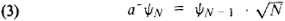Similarly, the operator of particle production, a+, transforms the state ψN into a state with N + 1 particles: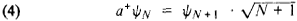The factors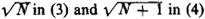are introduced just to satisfy the normalization condition |ψN|2 = 1. In particular, when N = 0, a+ψ0 = ψ1, where ψ0 is the state vector that characterizes the vacuum; that is, a single-particle state is produced as a result of the production of one particle from the “vacuum.” However, aψ0 = 0, since annihilation of a particle is impossible in a state in which no particles exist; this equation may be considered a definition of a vacuum. The vacuum state ψ0 has particular significance in quantum field theory, since any state can be derived from it by using a + operators. Indeed, in the case under examination (when the state of the entire system is determined only by the number of particles),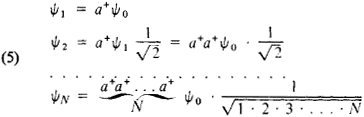It can be easily demonstrated that the order of action of the operators a and a+ is not immaterial. Indeed, a(a+ψ0) = aψ1 = ψ0, and a+(aψ0 = 0. Therefore, (aa+a+a0 = ψ0 or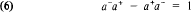that is, the operators a+ and a are noncommutative. Relations such as (6) that establish a connection between the action of two operators taken in different order are called transposition relations or commutation– relations for these operators, and expressions of the type Â̂B̂BÂ = [Â, ̂B] are said to be commutators of the operators Â and ̂B.

If it is taken into account that particles may exist in different states, then the state of the particle to which the operators refer must also be indicated in writing the production and annihilation operators. In quantum theory the states are given by a set of quantum numbers that define the energy, spin, and other physical quantities; for simplicity the whole set of quantum numbers will be designated by a single index n: thus, a+n designates the production operator for a particle in a state with a set of quantum numbers n. The average numbers of particles in the states corresponding to various n are called the occupation numbers of the states.

Let us examine the expression ana+m ψ0. First ψ0 is acted on by the operator “closest” to it, a+m this corresponds to the production of a particle in state m. If n = m, the subsequent action of the operator an leads again to ψ0 that is, ana+n, ψ00. If nm then ana+m ψ0 = 0, since the annihilation of particles that do not exist is impossible (the operator an describes the annihilation of particles in states n that do not occur when a+m acts on ψ0). With the different states of particles taken into consideration, the commutation relations for the creation and annihilation operators have the following form: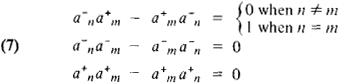However, fields exist for which the relation between the product of the production and annihilation operators taken in different order has the following form: the minus sign in (7) is replaced by a plus sign (this is called the replacement of commutators by anticommutators),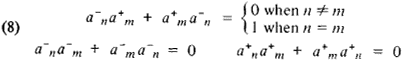These relations also apply to the class of commutation relations, although they do not have the form of (6). Operators that conform to relations (8) must be introduced for fields whose quanta have half-integral spin (that is, they are fermions) and as a result conform to the Pauli exclusion principle, according to which the existence of two or more particles in identical states (in states with identical sets of all quantum numbers) is impossible in a system of such particles (such as electrons). Indeed, by constructing the vector of a state containing two particles (a two-particle state), a+ it is not difficult to prove, taking (8) into consideration, that when n = m the vector is equal to itself with the opposite sign; but this is possible only for a quantity that is identically equal to zero. Thus, if the production and annihilation operators of particles satisfy the commutation relations (8), then states with two or more particles that have identical quantum numbers are automatically excluded. Such particles obey Fermi-Dirac statistics. However, for fields whose quanta have integral spin, the production and annihilation operators of particles satisfy relations (7); here states with an arbitrary number of particles having identical quantum numbers are possible.

The existence of two types of commutation relations is of fundamental importance, since it defines two possible types of statistics. The necessity of introducing noncommutative operators to describe systems having a variable number of particles is a typical feature of second quantization.

Let us note that “primary quantization” may also be considered as a transition from classical mechanics, in which the coordinates q and momentums p are ordinary numbers (that is, of course, qp = pq), to a theory in which q and p are replaced by noncommutative operators: q̂qp̂q̂p ≠, ̂p̂q. Here the transition from classical field theory to quantum field theory (as in electrodynamics, for example) is carried out by a similar method, but the role of the coordinates (and momentums) must be played by quantities that describe the field’s distribution throughout all space and at all instants of time. Thus, in classical electrodynamics a field is defined by the values of the intensity of the electric field E and the magnetic field H (as functions of the coordinates and time). When the transition to quantum theory is made, E and H become operators that do not commute with the operator of the number of photons in the field.

It has been proved in quantum mechanics that if two operators do not commute, the physical quantities corresponding to them cannot simultaneously have exact values. Hence it follows that no state of an electromagnetic field exists in which both the field intensity and the number of photons would be exactly defined. If the number of photons is known precisely by virtue of physical conditions, then the field intensities must be completely indeterminate (capable of assuming any values). However, if these intensities are known precisely, then the number of photons is indeterminate. Thus, the impossibility of simultaneously making the field intensities and the number of photons equal to zero is the physical reason why the vacuum state is not simply the absence of a field but retains important physical properties.

Field methods in the quantum theory of many particles. The mathematical methods of quantum field theory, as noted above, are used to describe systems that consist of a large number of particles: in solid-state physics, the physics of the atomic nucleus, and so on. The lowest energy states filled by a system (that is, at temperature T→0), for example, play the role of vacuum states in a solid. If energy is imparted to a system (for example, by increasing its temperature), it moves into an excited state. At low energies the process of excitation of a system may be considered to be the formation of certain elementary excitations—a process similar to the production of particles in quantum field theory. Certain elementary excitations in a solid behave like particles—they have a definite energy, momentum, and spin. They are called quasiparticles. The evolution of a system may be represented as the collision, scattering, annihilation, and production of quasiparticles. This opens the way for extensive use of the methods of quantum field theory. The theory of superconductivity is one of the most graphic examples of the fruitfulness of the methods of quantum field theory in the study of solids.

Quanta as carriers of interaction. In classical electrodynamics the interaction between charges (and currents) is accomplished through a field: the charge gives rise to a field, and the field acts on other charges. In quantum theory the interaction of a field and a charge takes on the form of the emission and absorption of field quanta (photons) by the charge. In quantum field theory an interaction between charges, such as two electrons, is a result of their exchange of photons: each of the electrons emits a photon (quanta transferring the interaction of the electromagnetic field), which is then absorbed by the other electron. This also is valid for other physical fields: in quantum field theory, interaction is a result of the exchange of field quanta.

However, this graphic picture of interaction has an aspect that requires additional analysis. Until the interaction begins, each of the particles is free, and a free particle can neither emit nor absorb quanta. Indeed, let us examine a free stationary particle (if a particle moves uniformly we may always transform to an inertial frame of reference, in which it is at rest). Such a particle has no stored kinetic or potential energy, so that radiation is energetically impossible. Somewhat more complicated arguments demonstrate that a free particle is also incapable of absorbing quanta. However, if these considerations are valid, then the seemingly inevitable conclusion is that the appearance of interactions in quantum field theory is impossible.

To resolve this paradox it is necessary to bear in mind that particles are quantum entities and that for them the uncertainty relations are effective. These relations relate the uncertainty of a particle’s coordinates (Δx) and its momentum (Δp):

(9) ΔxΔph/2

(where ℏ = h/2π). There is also a second relation—for the uncertainty of the energy Δε and the characteristic time Δt of a given physical process (that is, the time over which the process takes place):

ΔεΔtℏ ℏ

If the interaction between particles is considered to take place by the exchange of field quanta (this field often is called an intermediate field), then the duration of the exchange act is naturally taken to be Ar. The question of the possibility of emission of a photon by a free particle vanishes: according to (10), the particle’s energy is not precisely defined; when a quantum energy spread Δε is present, the laws of conservation of energy and momentum no longer impede either emission or absorption of the quanta transferring the interaction, provided they have approximately the energy Δε and exist for a time interval Δt ~ℏ/Δε.

The preceding arguments not only eliminate the paradox indicated above but also make it possible to draw important physical conclusions. Let us examine the interaction of particles in atomic nuclei. Nuclei consist of nucleons—protons and neutrons. Experiment has established that the interaction is imperceptible outside the boundaries of the nucleus, that is, at distances greater than approximately 10−13 cm, although the interaction is known to be great within the nucleus. It can therefore be accepted that the radius of action of nuclear forces is of the order of L ℏ 10−13 cm. Consequently, it is precisely this path that is traversed by quanta carrying the interaction between nucleons in atomic nuclei. The time spent by quanta en route, even if they are assumed to move at the greatest possible speed (the speed of light, c), cannot be less than ΔtL/c. According to the above discussion, the quantum energy spread Δε of interacting nucleons proves to be Δε ≈ ℏ/Δt ≈ ℏc/L. The energy of a quantum—the carrier of interaction—should also lie within this spread. The energy of every particle of mass m consists of its rest energy, equal to mc2, and its kinetic energy, which increases with the particle’s momentum. When the motion of particles is not too fast, the kinetic energy is small in comparison with mc2, so that we may say Δε ≈ mc2. Then it follows from the preceding formula that a quantum that carries interactions in the nucleus should have a mass of the order of mLc. If we insert in this formula the numerical values of the quantities, the mass of a quantum of the nuclear field is found to be approximately 200–300 times greater than the mass of the electron.

In 1935 this semiqualitative examination led the Japanese theoretical physicist H. Yukawa to predict a new particle: experiment later confirmed the existence of this particle, called the pi-meson. This brilliant result significantly strengthened faith in the correctness of quantum concepts of interaction as the exchange of quanta of the intermediate field, a faith that is largely retained to the present time, although so far it has been impossible to construct a quantitative meson theory of nuclear forces.

If we consider two particles so heavy that they can be regarded as classical material particles, the interaction between them arising as a result of the exchange of quanta of mass m leads to the appearance of a potential energy of interaction of the particles, equal to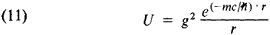where r is the distance between the particles and g is the coupling constant of the particles with the field of the quanta transferring the interaction (or, in other words, the charge corresponding to the given type of interaction).

If we apply this formula to a case in which the quanta of the electromagnetic field—photons, whose rest mass m = 0—are the carriers of the interaction, and if we take into account that the electric charge e should be used instead of g, we obtain the well-known energy of Coulomb interaction of two particles: Uel =e2/r.

Graphic method of describing processes. Although typically quantum objects are considered in quantum field theory, graphic representations of the processes of the interaction and conversion of particles may be given. Diagrams of this type were first introduced by the American physicist R. Feynman and bear his name. Feynman diagrams are outwardly similar to the representation of the paths of all particles participating in an interaction, if they were classical (although here no classical description whatever is involved). To represent every free particle, a line (which, of course, is only a graphic symbol of the particle’s propagation) is introduced: for example, a photon is represented by a wavy line, an electron by a solid line. Arrows that arbitrarily designate the “direction of propagation” of a particle are occasionally placed on the lines. Examples of such diagrams are given below.

A diagram corresponding to the scattering of a photon on an electron is presented in Figure 1: in the initial state there is one electron and one photon; at point (1) they collide and the photon is absorbed by the electron; a new, final photon appears (is emitted by the electron) at point (2). This is one of the simplest diagrams of the Compton effect.Figure 1

The diagram in Figure 2 illustrates the exchange of a photon between two electrons: one electron at point (1) emits a photon, which then is absorbed at point (2) by the second electron. As indicated above, an exchange of this type indicates that an interaction is present; thus, this diagram represents an elementary event of electromagnetic interaction between two electrons. More complicated diagrams corresponding to such an interaction should take into account the possible exchange of several photons; one of these is shown in Figure 3.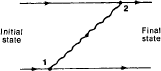Figure 2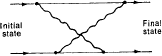Figure 3

In these examples a common property of diagrams that describe an interaction between electrons and photons is manifested: all diagrams are composed of extremely simple elements, or vertices, one of which (Figure 4) represents emission, and the other (Figure 5) represents absorption of a photon by an electron. Each of these processes separately is prohibited by the laws of conservation of energy and momentum. However, if such a vertex is a component of some more complicated diagram, as was the case in the examples considered above, then the quantum uncertainty of the energy, which results from the fact that in the intermediate stage a certain particle exists for a short time Ar, lifts the energy prohibition.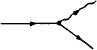Figure 4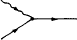Figure 5

Particles that are produced and then absorbed at intermediate stages of the process are called virtual particles (in contrast to real particles, which exist for a rather long period). In Figure 1 a virtual electron arises at point (1) and disappears at point (2), in Figure 2 it is a virtual photon, and so on. Interaction is often said to be transferred by virtual particles. It may be assumed on a somewhat tentative basis that a particle is virtual if the quantum uncertainty of its energy Δε is of the order of the average value of the particle’s energy ε, and it may be called a real particle if Δε «ε (for relatively slow-moving particles with nonzero rest mass m this condition reduces to the inequality Δε « mc2).

Feynman diagrams not only give a visual representation of processes but also make possible calculation of the probabilities of the processes by means of certain mathematical rules. Without dwelling on these rules in detail, let us note that an elementary interaction that leads to the conversion of particles (that is, to the annihilation of some particles and the production of others) occurs at every vertex. Therefore, each vertex contributes to the probability amplitude of the process, and this contribution is proportional to the interaction constant of the particles (or fields) whose lines meet at the vertex. In all of the diagrams presented above, this constant is the electric charge e. The more vertices the diagram of a process contains, the higher the power to which the charge enters the corresponding expression for the probability amplitude of the process. For example, the probability amplitude corresponding to the first and second diagrams, with two vertices, is the square of the charge (ℏ e2), and the third diagram, which contains four vertices, leads to an amplitude proportional to the fourth power of the charge (ℏe4). Moreover, at every vertex it is necessary to take into account the conservation laws (with the exception of the law of conservation of energy, whose applicability is limited by the quantum uncertainty relation for energy and time): the law of conservation of momentum (the interaction corresponding to each peak may take place at any point in space—that is, the uncertainty of the coordinate is Δx = ∞—and consequently the momentum is precisely defined) and the law of conservation of electric charge; factors that depend on the particles’ spin must also be introduced.

Only the simplest types of diagrams for certain processes were considered above. These diagrams do not exhaust the possibilities. Each of the very simple diagrams may be supplemented by an infinite number of increasingly complex diagrams that include an ever greater number of vertices. For example, the “lowest” diagram of the Compton effect presented in Figure 1 may be complicated by arbitrary selection of pairs of points on the electron lines and by joining these pairs with a wavy photon line (Figure 6), since the number of intermediate (virtual) photon lines is unlimited.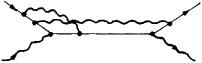Figure 6

Interaction of a particle with the vacuum electromagnetic field; atomic radiation. In the diagrams of the interaction of two electrons presented above (Figures 2 and 3), each photon is produced by one electron and absorbed by the other. However, another process is also possible (Figure 7): the photon emitted by an electron at point (1) is absorbed after a certain interval by the same electron at point (2). Since the exchange of quanta gives rise to an interaction, such a diagram also is one of the simplest interaction diagrams, but it is a diagram only of the electron’s interaction with itself or with its own field, which is the same thing. This process may also be called the electron’s interaction with a field of virtual photons or with a photon vacuum (the latter term is determined by the fact that here there are no real photons). Thus, the electron’s own electromagnetic (electrostatic) field is generated by the emission and absorption of photons by the same electron. Such interactions between an electron and a vacuum give rise to experimentally observable effects, attesting to the reality of the vacuum. The most significant of these effects is the radiation of photons by atoms. According to quantum mechanics, electrons in atoms occupy certain quantum energy levels, and the radiation of a photon takes place during the transition of an electron from one (the higher) level to another level that has less energy. However, quantum mechanics leaves open the question of why such transitions, which are accompanied by so-called spontaneous (“self-produced”) radiation, take place; moreover, each level here appears to be completely stable. According to quantum field theory, the interaction of the atom with the photon vacuum is the physical reason for the instability of excited levels and spontaneous quantum transitions. Figuratively speaking, interaction with the photon vacuum vibrates or rocks an atomic electron, since upon emission and absorption of every virtual photon the electron experiences a thrust or recoil; otherwise the electron would move stably in its orbit (for the sake of clarity, the semiclassical image is used). One such thrust forces the electron to “fall” into a more stable, or lower-energy, orbit; here energy is released and is used to excite the electromagnetic field—that is, to form a real photon.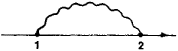Figure 7

The fact that the interaction of electrons with a photon vacuum accounts for the very possibility of transitions in atoms (and in other photon-radiating systems)—and hence for radiation—is the most far-reaching and significant effect in quantum electrodynamics. However, there also are other, much weaker “vacuum effects” that are very important in principle; some of them will be discussed later (see below: Perturbation method in quantum field theory).

Electron-positron vacuum. In 1928 the English physicist P. Dirac deduced the relativistic quantum equation of electron motion and from this equation predicted (1931) that the electron should have a “double”—an antiparticle differing from the electron in the sign of its electric charge. Such a particle, called the positron, was soon detected experimentally. A positron cannot be produced alone—this is precluded, for example, by the law of conservation of electric charge. Electrons and positrons may appear and disappear (be annihilated) only in pairs. A rather large quantity of energy (no less than twice the rest energy of the electron) is necessary for the production of an electron-positron pair. Such energy may be transferred, for example, by a “hard,” or high-energy, photon (a gamma-ray quantum) that strikes a charged particle. However, production of a pair may also occur virtually. In that case the pair that is formed is annihilated after having existed for some very brief time Δt. The quantum energy spread Δε ℏ t, if Δt is very small, makes this process permissible in terms of energy.

The process of production and annihilation of a virtual electron-positron pair is depicted graphically in Figure 8: the photon disappears at point (1), giving rise to a pair that is then annihilated at point (2), as a result of which a photon is formed once again. (The positron is represented by the same kind of solid line as the electron, on which line the arrow is, by convention, directed oppositely—that is, “backward” in time.)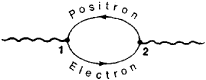Figure 8

The fact that electrons and positrons cannot appear and disappear separately but rather are produced and annihilated only in pairs indicates the genuine physical unity of the electron-positron field. Electron and positron fields appear to be isolated only until processes that involve a change in the number of electrons and positrons are considered.

Not only electrons have antiparticles. It has been established that every particle, except for so-called truly neutral particles such as the photon and the neutral pi-meson, has an antiparticle. Processes similar to the virtual production and annihilation of electron-positron pairs exist for any particle-antiparticle pair.

The mathematical and physical particle; field mass; renormalization of mass. The following method, which actually has already been used above, is frequently used to describe interacting fields. The quanta of free fields (particles) are considered first. This is the zero-order approximation, in which interaction is disregarded altogether. Then the interaction is brought into consideration—the particles cease to be independent, and the possibility of their scattering, production, and annihilation as a result of interaction appears. A successive increase in the number of interaction-induced processes that are taken into account is achieved mathematically by using the perturbation method. In view of the prominent role played by this method in theory, let us discuss its physical meaning in greater detail. The procedure of successive refinement of the contribution from interactions is actually used in classical electrodynamics as well. Let us explain this by using as an example the electron and the electromagnetic field that it generates. In theory the electron is the carrier of a certain mass m0. However, since it gives rise to an electromagnetic field with energy εel, and consequently (according to the relativistic relation ε = mc2) mass εel/c2, then on accelerating an electron it is also necessary to overcome the inertia of its electromagnetic field (in the simplest case, a Coulomb field).

Thus, in bringing into consideration the interaction between the electron and the electromagnetic field, the “field” part of the mass mfieldel/c2 must be added to the “nonfield,” or “bare” mass m0. Calculation of the field mass for a point particle (and the “bare” particles considered in the zero-order approximation must be considered point particles) leads to a physically meaningless result: mfield proves to be infinitely large. Indeed, the energy of the Coulomb field of a particle that has charge e and dimension a is equal to εCouiomb = ke2/a (k is a factor of the order of 1, whose numerical value depends on the charge distribution); as a→ 0 (point particle), £couiomb Coulomb→ ∞.

The infinite value (divergence) of field mass (although in somewhat altered, “weakened” form) is retained even in the transition from classical theory to quantum theory. Moreover, divergences of other types also appear. Analysis of the difficulties encountered here led to the emergence of the idea of renormalization. The division into field and nonfield mass arises (as can be seen from the preceding) because of the method of examination adopted: first a free “bare” particle is introduced, and then interaction is “switched on.” In experiments, of course, there is neither “bare” mass nor field mass, and only the total mass of the particle is manifest. In theory it is very significant that these masses also enter as a sum and not as separate quantities. Combination of field and nonfield mass and the use for total mass of a value derived experimentally rather than theoretically is called the renormalization of mass.

The traditional means of constructing a theory within the framework of the method of perturbation theory is as follows: first, a theory of free (noninteracting) particles is formulated, and then the interaction between them is introduced. Thus, the theory of free electrons (or of the electron-positron field) is constructed first, and then the interaction of these “mathematical,” or “bare,” electrons with the electromagnetic field is considered. However, the “physical” electrons that actually exist in nature, in contrast to “mathematical” electrons, always interact with photons (although only with virtual photons), and this interaction may be “canceled out” only in theory. An important part of the idea of renormalization is the indication of the necessity of constructing a theory in which not the mathematical but rather the physical particles play a role.

It is interesting that to some extent nature provides the possibility of seeing the difference between a particle with “switched on” and then “switched off’ electromagnetic interaction. For example, three pi-mesons are known: with a positive electric charge (π+), a negative charge (π) and a zero charge(π0). They are different charge states of the same particle. Charged mesons(π+ and π) have a greater mass than the neutral meson (π0); obviously, an addition caused by the field (electromagnetic) mass is manifested here, although theory is not yet able to provide an entirely clear quantitative explanation of this phenomenon.

The process of the “clothing” of a mathematical particle— that is, its conversion into a physical particle—appears more complex in quantum field theory than in classical electrodynamics, where everything reduces to the “attachment” of a Coulomb “tail” to the particle. In quantum theory a physical particle differs from a mathematical particle by its “coat,” which is much more complex in structure: it is formed by “clouds” of virtual quanta that are produced and then absorbed by the particle. They may be quanta of any of the fields with which the particle is interacting (such as an electromagnetic, electron-positron, or meson field). The “coat” is not something fixed; the quanta forming it are created and absorbed continuously. The “coat” pulsates—that is, the particle carrying it spends part of its time in the “clothed” state and part in the “bare” state. The part of its time that is spent in each state is determined by the degree of intensity of the interactions. For example, the meson interactions of nucleons are more than 100 times more intense than their electromagnetic interactions; one may assume that the meson “clothing” of a proton is more than 100 times “denser” than the electromagnetic. This may explain why the quantum theory of electromagnetic processes agrees brilliantly with experiment, even when vacuum effects are far from completely taken into consideration, whereas meson theory has achieved no such success. In quantum electrodynamics we may limit ourselves to consideration of processes with a small number of virtual photons and virtual electron-positron pairs, corresponding to consideration of a small number of “lower order” corrections according to the method of perturbation theory; in meson theory this does not lead to success, generating difficulties that will be examined in the next section (see below: Difficulties and problems in quantum field theory).

All the reasoning concerning the “coat” of particles presented above is, strictly speaking, semi-intuitive and cannot yet be translated into the language of exact theory. However, it may be useful if only because it helps to clarify the distinction between mathematical and physical particles and to understand that the description of the latter is not at all a simple task.

Polarization of a vacuum; renormalization of charge. The electric, and above all the Coulomb, field of a charged particle affects the distribution of virtual electron-positron pairs (and of pairs of any other charged particles and antiparticles). A real electron attracts virtual positrons and repels virtual electrons. This should lead to phenomena resembling the polarization of a medium into which a charged particle is introduced. Once again the perturbation theory is applicable for describing such phenomena.

The polarization of an electron-positron vacuum (use of the term suggested by the analogy cited above is accepted) is a purely quantum effect that derives from quantum field theory. The polarization leads to surrounding of the electron by a dense layer of positrons from virtual pairs, so that the effective charge of the electron must be changed significantly. Shielding of the charge, or effective reduction of the charge, results. If “bare” particles are considered as point particles, then the screening is complete —that is, the effective charge is a zero charge (the “zero charge” problem). The idea of renormalization of charge is used to overcome this difficulty. Here the arguments cited during the discussion of mass renormalization are repeated almost verbatim. Let us call “bare” a charge that would be held by a particle if the interaction with the electron-positron vacuum disappeared (we will speak only of such a particle, although, of course, the influence of virtual pairs of other fields must also be taken into account). The existence of such interaction leads to the appearance of a “correction” of the charge. Physicists are unable to calculate this correction correctly, just as they cannot determine a “bare” charge. However, since these two parts of a charge do not act alone either in experiment or in theory, the difficulty can be avoided by inserting in place of the total charge a quantity taken directly from experiment. This procedure is called renor-malization of charge. Renormalization of charge and mass does not solve the problems that arise in the theory of point particles but rather only isolates these problems at some stage of theory and—extremely importantly—provides the possibility of isolating finite observable parts from infinite values for certain quantities that characterize physical particles.

Some observable “vacuum” effects. The possibility of observing the influence of a “vacuum’’ on particles exists. The “coat” of physical particles is found to depend on the external fields acting on the particle. In other words, field additions to a particle’s energy depend on its state. The total field energy, as stated above, is infinitely large in the theory of point particles, but a finite part that changes as a function of the state of the particle and therefore can be detected experimentally can be isolated from this infinitely large quantity.

LAMB SHIFT. In the hydrogen atom (and some other light atoms) there are two states, 2S1/2 and 2P1/2, whose energies— according to quantum mechanics—should coincide. At the same time, the pattern of the motion of electrons in these states is different. Figuratively speaking, an. S-electron (an electron in the 5-state) spends most of its time near the nucleus, and a P-electron is located, on the average, at a greater distance from the nucleus. Therefore, on the average, an. S-electron is in a stronger field than a P-electron. This leads to differences in the additional energy caused by the interaction with the photon vacuum for the P-electron and the S-electron; this may be clarified graphically. As stated above, interaction with the vacuum jolts the electron. Instead of moving in some stable orbit, such as a circular orbit, of radius r (we shall once again use this classical picture), the electron begins to be deflected at random away from this orbit. When the deflection in each direction is Δr the energy changes in different ways. Indeed, the Coulomb energy of an electron in a nuclear field changes according to the law ɛpotential ℏ 1/r as r increases by Ar the energy changes by the amount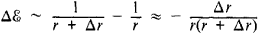and as r decreases by Δr, the energy changes by the amount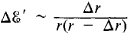that is, the absolute value of Δε′ is greater than that of Δε. As a result, the “vacuum jitter” of the electron changes the value of its potential energy. The change is particularly noticeable where the potential energy itself is great and changes rapidly with a change in r—that is, close to the nucleus. Thus, the vacuum additions to energy, called radiation corrections, should be greater for P-electrons than for P-electrons, and their energy levels, which otherwise would coincide, should split. The extent of the splitting, called the Lamb shift (it was first explained theoretically by H. Bethe and observed experimentally in 1947 by the American physicists W. Lamb and R. Retherford), is, according to quantum field theory, equal to the following (if expressed in units of frequency v): 1057.77 MHz for hydrogen, 1058.9 MHz for deuterium, and 14, 046.3 MHz for helium (conversion to energy units, ergs, is carried out according to the formula ε = hv, where v is expressed in hertz). These values are in such good agreement with experimental data that further improvement of experimental accuracy would lead to the detection of effects caused by so-called strong interactions rather than electromagnetic interactions.

ANOMALOUS MAGNETIC MOMENT. No less remarkable is the accuracy with which the anomalous magnetic moment of the electron, which also shows “vacuum” (radiation) influences on this particle, is calculated. it follows from Dirac’s quantum theory of the electron that the electron should have magnetic moment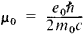However, this applies to the “bare” electron. The process of “clothing” it changes the magnetic moment. In bringing the interaction between the electron and the vacuum into the discussion, it is necessary first of all to replace the charge (e0) and mass (mo) of an idealized mathematical particle with the physical values of these quantities:

mmphys. ℯ → ℯphys.

However, this does not exhaust the observed effects. The magnetic moment is a quantity that conditions the interaction between a stationary particle and an external magnetic field. It is natural to interpret the corrections that appear in the expression for the energy of the interaction as a result of the appearance of “vacuum” additions to the magnetic moment. These additions, first studied theoretically by J. Schwinger, give the so-called anomalous magnetic moment. The anomalous magnetic moment of the electron has been calculated and measured with high precision, as shown by the following data: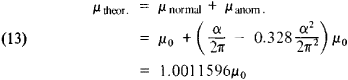where α is the fine structure constant, equal to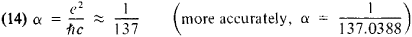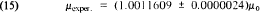Here again the striking coincidence of the measured magnetic moment of the electron and the value obtained on the basis of quantum field theory is observed.

SCATTERING OF LIGHT BY LIGHT. There are other effects described by quantum field theory. We shall limit ourselves to examining one more effect predicted by quantum field theory. The principle of superposition is known to be valid for electromagnetic waves: upon superposition, electromagnetic waves do not have any effect on each other. This principle of the superposition of waves without mutual distortions passes from classical theory into quantum theory, where it assumes the form of the assertion that there is no interaction between photons. However, this situation changes if the effects caused by the electron-positron vacuum are taken into account.

The diagram presented in Figure 9 corresponds to the following process: In the initial state there are two photons; one of them disappears at point (1), creating a virtual electron-positron pair; and the second photon is absorbed by one of the particles of the pair (in this diagram, by the positron) at point (2). Then final photons appear: one of them is produced at point (3) by the virtual electron, and the other arises as a result of the annihilation of the pair at point (4). This diagram (and an infinite number of other, more complicated diagrams) shows that interaction between photons must appear as a result of virtual electron-positron pairs, that is, the principle of superposition must be violated. Violations should be manifested in such processes as the scattering of light by light (however, this effect is so slight that it has not yet been observed experimentally). The process of scattering of photons in an external electrostatic field, which has a somewhat higher probability, as yet lies outside experimental capabilities, but the successes of quantum electromagnetics have been so great that there can be no doubt as to the adequacy of these predictions.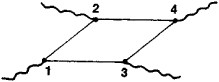Figure 9

In addition to these effects, “higher” corrections that are calculated on the basis of the perturbation method (radiation corrections) appear in the process of the scattering of charged particles and in some other phenomena.

A success requiring explanation. The advances of quantum electrodynamics discussed above are impressive but not fully explicable. These advances are associated with the analysis of only the simplest, lowest Feynman diagrams, which take into account only a small number of virtual particles or, in mathematical language, low-order approximations of perturbation theory. In considering higher approximations, an infinite number of increasingly complex diagrams of higher orders that include an ever greater number of internal lines, each of which corresponds to a virtual particle, could be added to each diagram. It is true that an increasingly large number of vertices will be found in such complicated diagrams, and each vertex introduces into the expression for the probability amplitude of the process a factor e, or more accurately,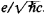. Since the internal lines have two ends (two vertices), the addition of each internal line changes the amplitude by a factor of approximately e2/ℏ ≈ 1/137. If the amplitude is written as the sum of terms with ascending powers of the quantity α = e2/ℏc (the construction of this sum, or series, corresponds mathematically to an application of the method of perturbation theory), then a Feynman diagram with an increasingly large number of internal lines will correspond to each successive term. Therefore, every term of the series should be approximately two orders of magnitude (100 times) smaller than the previous one. Higher diagrams would indeed seem to provide a negligible contribution and can be discarded. However, more careful examination shows that, since the number of such discarded diagrams is infinitely large, assessment of their contribution is neither simple nor obvious. The task is complicated still further, since α acts in combination with a factor proportional to the logarithm of the energy, so that at high energies the perturbation method proves to be ineffective.

Although this problem may not seem very pressing in quantum electrodynamics, since here theory brilliantly describes experiment, it is quite a different matter in the theories of other fields.

Problem of strong interactions. The theory of strong interactions began to develop by analogy with quantum electrodynamics, but the role of carriers of interaction was ascribed, as indicated above, to pi-mesons—particles that have a rest mass approximately 200 times greater than the rest mass of the electron. However, a circumstance that fundamentally distinguishes electrodynamics from meson dynamics was revealed here: the interaction constant g —that is, the quantity that plays the role of the charge in strong interactions—is relatively large, and in meson dynamics the quantity g2/ℏc > 1 appears instead of e2/ℏc ≈ 1/137 «1. Therefore, the arguments that in electrodynamics to some extent justify the discarding of higher diagrams (that is, the use of lower approximations of perturbation theory) lose their force in meson dynamics. It is not surprising that, in the case of strongly interacting particles, consideration of only lower diagrams does not give agreement with experimental results. In other words, the perturbation method is inapplicable here for calculation of the probability amplitude.

A peculiar situation has taken shape in quantum field theory: equations for interacting fields were written many years ago, and in principle a method has been found to single out that which corresponds to physical particles, but at the same time theoreticians do not know how to solve the equations exactly. Approximate methods, above all the method of perturbation theory, are not always suitable. However, without knowing the exact solution to the equations of quantum field theory, it is difficult to assess with confidence the validity of these equations and, hence, the physical concepts on which they are based.

The difficulties in solving the equations of quantum field theory do not give rise only to “technical” problems. The method of solution largely determines the physical images with which theory operates. For example, what are the “mathematical” particles and the procedure for “clothing” them that were discussed above? All these concepts were dictated by perturbation theory: in the zero-order approximation the interaction is not taken into account at all (hence the term “bare” particles), and in subsequent approximations interaction is taken into account by introducing one, two, or more virtual particles; thus arises the picture of the gradual “envelopment” of a particle by a cloud of virtual quanta. However, in nature there are no “mathematical” particles: all particles are “physical,” and they are the ones that theory must describe. Although precisely this task is set in renormalization theory, specific calculations force a return to perturbation theory (let us note that in electrodynamics the fundamental possibility of carrying out renormalizations in any approximation is proved).

Problem of renormalizability; analysis of theoretical difficulties. Before the appearance of the idea of renormalization, quantum field theory could not be considered as a self-consistent concept, since meaningless, infinitely large values (divergences) appeared for some physical quantities and there was no understanding of what should be done with them. The idea of renormalization not only explained the observed effects but at the same time imparted to the entire theory features of logical completeness, eliminating the divergences.

Figuratively speaking, a method was proposed for taking into account changes in the “coat” of physical particles, depending on external conditions, and of quantitatively studying related effects. At the same time the “clothing” of the particle itself drops out of consideration. The particle is considered as a whole in its external manifestations—that is, in its interaction with other particles.

The renormalization program can by no means always be carried out successfully (that is, the renormalization of a finite number of quantities eliminating divergences). In some cases the consideration of diagrams of increasingly high order leads to the appearance of divergences of new types—then the theory is said to be nonrenormalizable. The first versions of the theory of weak interactions are examples of such a theory. Entities whose internal structure is manifested in their interactions may be encountered in nonrenormalizable theories.

Thus the perturbation method, in which the concept of free fields is used as the starting point and then the increasingly complex picture of interactions is examined, proves to be effective in quantum electrodynamics, since in this theory results that agree well with experiment can be obtained by means of renormalizations. However, even in this theory the problem of divergences cannot be considered to have been solved (the divergences are merely isolated, rather than eliminated). In other theories the situation is still more intricate. For example, in the theory of strong interactions the perturbation method ceases to be applicable. Thus, there are indisputable fundamental difficulties in quantum field theory that have not yet been solved.

There are several trends in explaining the reasons for the appearance of difficulties. According to one viewpoint, all complications are due to an incorrect method of solving the equations of quantum field theory. Indeed, the perturbation method has obvious shortcomings; moreover, it gives rise, for example, to the problem of renormalizations. If Heisenberg’s picture is used to describe fields, the necessity of introducing “mathematical” particles and of examining their subsequent “clothing” can be avoided. Here the only particles that figure in theory are “physical.” However, in order to introduce such particles it is necessary to assume that all interactions begin at some—although perhaps very remote—instant and then cease in the future, which also may be very distant. This concept is actually close to what occurs in an experiment, where an interaction begins when some particles strike other target particles and the products formed on collision fly so far apart after some period of time that interaction between them ceases. However, the possibility of asymptotic consideration of free fields (that is, at the instants t = − ∞ and t = + ∞)—and, consequently, of particles—does not remove all difficulties, since entirely effective methods of solving the equations for Heisenberg operators have not yet been found. Thus, according to this point of view, the reason for the difficulties lies precisely in the inability to solve the equations of quantum field theory with sufficient accuracy.

Also widespread is the opinion that, after ridding itself of all shortcomings of the perturbation method, theory will not attain the desired perfection—that is, the difficulties are not mathematical but physical in nature. It is pointed out, for example, that the consideration of a limited number of types of interacting fields is incorrect, since all fields are interconnected. Possibly the consistent consideration of all fields in their interaction (including the gravitational field) will lead to a correct and noncontra-dictory description of phenomena.

Reexamination of the concepts of interaction is also typical of the nonlocal quantum field theories that proceed from the assumption that the interaction among fields is “blurred,” that is, is not determined only by the values of the fields at a single point in space and at identical moments in time. The requirements of the theory of relativity impose extremely rigid limitations on the possible types of “blurring,” leading in particular to the emergence of the problem of causal description in nonlocal theories.

In another trend, the reason for the complications is that modern theory tries to give an excessively detailed description of phenomena in the microcosm. Just as such classical concepts as the trajectory of a particle and the tracking of its coordinates at all subsequent moments in time lose meaning after the transition from classical mechanics to quantum mechanics, it is impossible (and wrong) to try to describe in accepted concepts a detailed picture of the evolution of a field over time—it is possible only to raise the question of the probability of transition from the initial states of the field, when interaction has not yet begun, to the final states, when it is already ended. The task is to find laws that define the probabilities of such transitions (let us note that such a program actually goes beyond the framework of traditional quantum field theory). Here the operator (called the S-matrix) that establishes the relation between the state vector ψ(− ∞), in the infinite past (t = − ∞), and the vector ψ(+∞), which refers to the infinite future (t = + ∞), ψ(+∞) = Sψ(− ∞), takes on the greatest importance. The problem is to find laws that would determine the S-matrix but not based on a detailed description of the evolution of the system at all intermediate times between t = − ∞ and t = + ∞. studies based on an examination of the dependence of the S-matrix on the charge and leading to new types of solutions to problems of quantum field theory may, for example, attest to the possibilities that open up here.

Finally, we must not fail to mention another widely held opinion, which states that to eliminate theoretical flaws a radical step is needed—that is, a fundamentally new idea, as a result of which a new universal constant, such as a fundamental (elementary) length, is introduced. Repeated attempts at reexamining the concepts of space and time and attempts that use the concept of such a fundamental length have already been made.

Analysis of the reasons leading to the appearance of difficulties in theory is of great importance, but new ways of developing theory play no less a role. Some of them are examined below.

The “axiomatic approach” is one important example of a new approach to the study of quantum fields. Careful analysis of the assumptions that make up the mathematical and physical foundations of theory and the isolation of the most “reliable” assumptions are typical of this approach. Among the postulates (axioms) are relativistic invariance (that is, satisfaction of the requirements of the theory of relativity); the condition of causality, or of the localizability of interaction, which leads to the requirement for commutativity of field operators referring to different points in space and to moments in time that exclude the possibility of exchanging signals at a speed exceeding the speed of light (the exclusion of signals traveling faster than the speed of light corresponds to the requirement that cause always precede effect in time); and the condition of the so-called spectral character, which means the requirement that the energy of all permissible states of a physical system (the energy spectrum) be positive if the energy of the vacuum state is taken as zero. The question of the possibility of producing experimentally verifiable predictions pertaining to interacting fields on the basis of the adopted axioms is very important. The understanding of whether a self-consistent theory of such fields can be constructed on this basis is no less important.

One of the reasons for interest in the axiomatic approach is that it should indicate effects that are accessible to experimental study and that stem from current concepts of space and time and thus should make possible direct verification of these concepts. For example, experiments in which a violation of the axiom of localizability is detected would serve as proof of the necessity of revising the physical picture of space-time at extremely short distances.

The CIT theorem is a very important example of what can be derived from the fundamental postulates of quantum field theory. From the condition of localizability and relativistic invariance it follows that a theory must be invariant with respect to three simultaneously performed operations: spatial inversion I (the replacement of the coordinates r by − r), time inversion I (replacement of the time r by − r), and charge conjugation C (the replacement of particles by antiparticles); more graphically, the CIT theorem is formulated as an assertion of the invariance of theory with respect to the replacement of the incident particles by the emerging antiparticles in any process. The nontrivial nature of the CIT theorem is apparent if only from the fact that, for example, in weak interactions there is no invariance only with respect to spatial inversion and/or charge conjugation.

There is yet another peculiarity of the axiomatic approach: the careful studies conducted within its framework make possible detection of the initial assumptions in traditional quantum field theory that require logical and mathematical refinement.

The intensive development of the technology of charged-particle accelerators and the resulting unprecedented increase in the flow of experimental information on elementary particles have had an appreciable effect on the direction of theoretical inquiries. Particular attention is directed to a quantity that has a direct physical meaning—the scattering amplitude (the square of its modulus defines the probability of a process). For every process a diagram resembling a Feynman diagram but with a fundamentally different meaning may be correlated with the scattering amplitude. Let us examine, for example, the diagram depicted in Figure 10. It is similar to a graph of the vertex part (see Figures 4 and 5)—and is called a vertex diagram—but it is not a graphic representation of an approximate solution (obtained by means of perturbation theory) to some equation; the graph simply records a process in which the particles A, B, and C take part. If the mass mA of a particle A is greater than the sum of the masses mB + mc of particles B and C, the diagram describes the real decay A → B + C If decay is prohibited in terms of energy, at least one of the lines in the diagram refers to a virtual particle. The circle in Figure 10 means that the vertex is physical—that is, it corresponds directly to what occurs in experiment. If the lines A and B refer to real nucleons (such as protons) and line C stands for a virtual photon, then the vertex part depends only on a single variable. The requirements of the theory of relativity force the selection of the quantity Pc2 = εc2/c2 — Pc2 as such a variable, since only this combination of the energy εc and the momentum pc of the particle does not change upon transition from one inertial reference system to another; the quantity pc is called the four-dimensional momentum of the particle C. For a real particle Pc2 = mc2, and the particle is said to be on a mass surface. Virtual particles lie “outside the mass surface”; this is due to the presence of a noticeable quantum energy spread or a quantum mass spread, which is equivalent.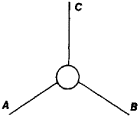Figure 10

The dependence of the scattering amplitude on pc2 describes the experimentally observed distribution of electric charge, magnetic moment, and all higher electric and magnetic multipole moments of the proton (the electromagnetic form factor of the proton). Within the framework of the methods that were discussed above and are typical of quantum electrodynamics, such a form factor should, in principle, be sought by analyzing the proton’s “coat”; as noted above, effective methods for such an analysis do not exist. An important feature of the new approach is the use of experimental data to fill gaps in the theory.

Let us cite yet another important example of “generalized” diagrams—the “four-tail” diagram (Figure 11). It represents either the decay of one particle into three (A → B + C + D), if the process is allowed in terms of energy, or transitions of the type in which two particles yield two particles, in particular if the particles are identical at the start and end of the process (elastic scattering of the particles). Let us examine this latter process and, for the sake of simplicity, assume that all the particles have identical mass and zero spin. Then, if all four lines refer to real particles, the scattering amplitude proves to be dependent only on two invariant variables. The following variables are usually used: s = (pA + pB)2, which is equal to the square of the energy of the colliding particles in the center-of-momentum system (that is, in a reference system in which the total momentum of particles A and B is equal to zero), and t = (pA +Pc)2 which defines the momentum transfer upon scattering.

The diagrams presented in Figures 10 and 11 do not, of course, exhaust all possibilities. However, they play a significant role and often are used as “units” for the construction of more complicated diagrams that describe processes involving a larger number of particles (more than four).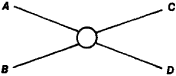Figure 11

The apparatus of the theory of analytic functions is used in studying the scattering amplitude f. Here s and t, on which the scattering amplitude f(s, t) depends, are considered to be complex variables. This approach is justified by the fact that the behavior of analytic functions is determined largely by the type and position of the singularities of the function. One of the most important types of singularity is the pole of the function f(z) at some point z0, which corresponds to the value of the function I at this point approaching infinity as l/(zzo). The poles in the scattering amplitude can be given a descriptive interpretation. For example, if a pole of the type 1/(sm2c4) appears in the scattering amplitude that describes the process A + B → C + D, this means that the process is taking place through an intermediate (virtual) particle Q, A + B → QC + D, and the mass of the intermediate particle is mQ = m. A pole of the type 1/(tm2c4) corresponds to the diagram presented in Figure 12; m is the mass of the intermediate (virtual) particle on the diagram. The peculiarities of other types also may be interpreted physically as a reflection of certain important processes that are manifested at intermediate stages of scattering. If all these singularities are found, then we may try, on the basis of the general theorems of the theory of analytic functions, fully to recover the form of the scattering amplitude for all values of s and t and in particular for the real values of these quantities that are of direct interest to physicists. The fundamental principles of relativistic quantum mechanics mentioned above, as well as a number of others, are used to find the singularities. An important role is played by the condition of unitarity, which means that if a process may take place in several ways (if it may take place through different “channels”), for example,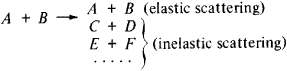then the total probability of all possible conversions is equal to unity. Despite their apparent triviality, such requirements as the unitarity and positive nature of the energies of physical particles place very strict limitations on scattering amplitudes.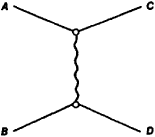Figure 12

The requirements of symmetry, in particular the fact that particles can be divided into groups, within each of which the masses increase in direct proportion to the spins, also play a very important role in constructing the scattering amplitude for various processes. Finally, it is necessary to take into account the laws of conservation that are important for each of the specific processes under consideration (such as the laws of conservation of electric charge, baryon charge, and lepton charge).

Quantum field theory also makes successful use of some methods that first appeared in classical electrodynamics. One of them is the method that shows the relation between the frequency-dependent real and imaginary parts of the dielectric constant of a dielectric. Since the dependence of the index of refraction of a dielectric on the frequency of the light is called dispersion (and the index of refraction is defined by the dielectric constant), this connection is called the dispersion relation. It was found that even without making any specific assumptions about the structure of the dielectric it is possible on the basis of the requirement of causality, which here assumes the form of the requirement that the polarization of a dielectric at any moment be determined only by the intensities of the electric fields at the same or at previous moments (but not at subsequent moments), to obtain an expression for the imaginary part of the dielectric constant, which determines the absorption of the electromagnetic wave, if its real part is known throughout the entire infinite range of frequencies (and vice versa). Dispersion relations make it possible to draw conclusions that can be verified by direct experiment, for example, the conclusion that dispersion is normal in regions of transparency (that is, at frequencies corresponding to low absorption): the index of refraction increases with frequency. In addition, information on the asymptotic behavior of the real and imaginary parts of the dielectric permeability (at very high frequencies) can be obtained from dispersion relations.

Since the classical problem of dispersion, or the scattering of electromagnetic waves in matter, is solved within the framework of the dispersion approach without using any specific models of the structure of matter, it is natural to expect that this approach also will prove productive in an examination of other problems of scattering, and particularly in quantum field theory. Here we may also separate the real and imaginary parts of scattering amplitude (the latter of which reflects the contribution of inelastic processes during which new particles appear in the final state) and to establish relations between them. The imaginary part of the scattering amplitude takes into account all possible processes (including elastic processes). The optical theorem asserts that the imaginary part of the forward scattering amplitude is proportional to the total probability of scattering.

The dispersion approach, which found a reliable mathematical basis and development in the works of N. N. Bogoliubov and his school, has made possible the derivation of a number of interesting results. Among them are the determination of precise values of the interaction constants for pi-mesons with protons and neutrons (nucleons), and also the interaction constants for kaons, nucleons, and λ-hyperons. Predictions with respect to the asymptotic behavior of scattering amplitudes are also of considerable interest.

However, the program for the complete construction of the amplitudes of processes within the framework of the dispersion approach has not yet found a final solution. Obviously, in addition to the general principles discussed above, the theory should rely on some more specific assumptions that play the role of dynamic principles. Sometimes such new dynamics assumes the form of an indication of the rules according to which the singularities of amplitudes should be determined; finding these rules requires careful use of experimental data. However, such “indirect” consideration of dynamics is not the only possible approach.

We cannot help but comment on the revival of interest in theories in which the laws of dynamics once again assume the traditional form of equations that describe a detailed space-time picture of processes. Important studies of the systematics of elementary particles and the establishment of new symmetry properties have been the impetus for this interest. It is natural to seek dynamic laws behind the principles observed here. The very interesting, although preliminary, results of attempts to coordinate field dynamics with the symmetry properties of elementary particles apparently are leading to the necessity of examining nonlinear, or self-stressing, fields. In one sense this trend is close to a unified quantum field theory, in which attempts are made to consider matter as a whole as a unified fundamental field (or as several basic types of fundamental fields) and individual particles as different manifestations (states) of the field.

An assessment of all current attempts to solve the problems that are arising in quantum field theory would be premature. However, the very fact that such attempts are so numerous attests to the gravity of the problems and to the efforts that are being made to answer the main question of physics—the question of the structure of matter.

### REFERENCES

Landau, L. D., and E. M. Lifshits. Teoriiapolia. Moscow, 1967. (Teoreticheskaia fizika, vol. 2.)
Schweber, S. Vvedenie v reliativistskuiu kvantovuiu teoriiu. Moscow, 1963. (Translated from English.)
Bogoliubov, N. N., and D. V. Shirkov. Vvedenie v teoriiu kvantovannykh polei. Moscow, 1957.
Salam, A. “Fundamental’naia teoriia materii (rezul’taty i metody).” Uspekhi fizicheskikh nauk, 1969, vol. 99, fasc. 4, pp. 571–611.
Akhiezer, A. I., and V. B. Berestetskii. Kvantovaia elektrodinamika, 3rd ed. Moscow, 1969.
Ziman, J. Sovremennaia kvantovaia teoriia. Moscow, 1971. (Translated from English.)
Bogoliubov, N. N., I. T. Todorov, and A. A. Logunov. Osnovy aksiomati-cheskogo podkhoda v kvantovoi teorii polia. Moscow, 1969.
Eden, R. Soudareniia elementarnykh chastits pri vysokikh energiiakh. Moscow, 1970. (Translated from English.)
V. I. GRIGOR’EV

## quantum field theory

[′kwän·təm ′fēld ‚thē·ə·rē]
(quantum mechanics)
Quantum theory of physical systems possessing an infinite number of degrees of freedom, such as the electromagnetic field, gravitation field, or wave fields in a medium.
McGraw-Hill Dictionary of Scientific & Technical Terms, 6E, Copyright © 2003 by The McGraw-Hill Companies, Inc.
References in periodicals archive ?
Moreover: those quantum field theories, which are technically all gauge theories with spontaneously broken symmetries, are extraordinarily successful as the standard model of particle physics has demonstrated.
In this context a conceptual 'crisis' could be seen in the dilemma that the best available theory of space-time, the general relativity theory, refuses--in a hitherto unsolvable manner--to be combined with the quantum field theories, which are constructed to describe the complete matter and energy content of the space-time, and do so excellently as far as the known forms of matter and energy are concerned.
In these studies, classical or quantum field theories are defined and characterized after replacing the ordinary Minkowski background with some noncommutative algebra of coordinates.
Frobenius algebras and 2D topological quantum field theories.
Kock covers cobordisms and topological quantum field theories, Frobenius algebras, monoids and monoidal categories, under the theorem that 2D topological field theories are the same as commutative Forbenius algebras.
Similarly quantum field theories have fields built upon a possible (historical) spacetime, and subjected to quantisation.
There we arrived at a HQS by deconstruction of the functional integral formulation of quantum field theories retaining only those structures which we felt would not be emergent.
Of these, noncommutative quantum field theories have motivated a great interest [12-17].
Hossenfelder, "Interpretation of quantum field theories with a minimal length scale," Physical Review D, vol.
This model is simple enough to be analyzed with full details; and yet it contains many of the typical features of the quantization of relativistic quantum field theories .
It is well known that instantons are classical topological solutions in quantum field theories which lie behind the standard model of particles.
A characteristic feature of continuum quantum field theories, which are used in high-energy physics, is ultraviolet divergences.

Site: Follow: Share:
Open / Close